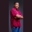Related Tags

number
c#
octal conversion
string to octal
communitycreator

# How to convert a number string to an octal in C#Theodore Kelechukwu Onyejiaku

### Overview

We can use the method Convert.ToInt() method in C# to convert a number string to an octal value. For example, we can use this method to convert the number string 30 to a base number of 8, which is an octal value.

### Syntax

Convert.ToInt32(numberString, 8)
Converting a number string to an octal in C#

### Parameters

numberString: This is the number string that we want to convert to an octal value.

### Return value

The value returned is an octal equivalent to the number string.

### Code example

using System;
class HelloWorld
{
static void Main()
{
// Creating some number strings
string num1 = "34";
string num2 = "1";
string num3 = "222";

// Converting to Octal and printing the results
Console.WriteLine("{0} {1}", num1, Convert.ToInt32(num1, 8));
Console.WriteLine("{0} {1}", num2, Convert.ToInt32(num2, 8));
Console.WriteLine("{0} {1}", num3, Convert.ToInt32(num3, 8));
}
}
Converting a number string to an Octal value

### Explanation

• Lines 7–9: We create some number strings.
• Lines 12–14: We convert the number strings to octal values using the Convert.ToInt32() method. Then, we print the values to the console.

RELATED TAGS

number
c#
octal conversion
string to octal
communitycreator

CONTRIBUTORTheodore Kelechukwu Onyejiaku
RELATED COURSES

View all Courses

Keep Exploring

Learn in-demand tech skills in half the time